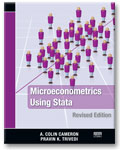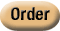»  Home »  Resources & support »  Announcements »  Microeconometrics Using Stata, Revised Edition

Microeconometrics Using Stata, Revised EditionMicroeconometrics Using Stata, Revised Edition, by A. Colin Cameron and Pravin K. Trivedi, is an outstanding introduction to microeconometrics and how to do microeconometric research using Stata. Aimed at students and researchers, this book covers topics left out of microeconometrics textbooks and omitted from basic introductions to Stata. Cameron and Trivedi provide the most complete and up-to-date survey of microeconometric methods available in Stata. The revised edition has been updated to reflect the new features available in Stata 11 that are germane to microeconomists. Instead of using mfx and the community-contributed margeff commands, the revised edition uses the new margins command, emphasizing both marginal effects at the means and average marginal effects. Factor variables, which allow you to specify indicator variables and interaction effects, replace the xi command. The new gmm command for generalized method of moments and nonlinear instrumental-variables estimation is presented, along with several examples. Finally, the chapter on maximum likelihood estimation incorporates the enhancements made to ml in Stata 11. Early in the book, Cameron and Trivedi introduce simulation methods and then use them to illustrate features of the estimators and tests described in the rest of the book. While simulation methods are important tools for econometricians, they are not covered in standard textbooks. By introducing simulation methods, the authors arm students and researchers with techniques they can use in future work. Cameron and Trivedi address each topic with an in-depth Stata example, and they reference their 2005 textbook, Microeconometrics: Methods and Applications, where appropriate. The authors also show how to use Stata’s programming features to implement methods for which Stata does not have a specific command. Although the book is not specifically about Stata programming, it does show how to solve many programming problems. These techniques are essential in applied microeconometrics because there will always be new, specialized methods beyond what has already been incorporated into a software package. Cameron and Trivedi’s choice of topics perfectly reflects the current practice of modern microeconometrics. After introducing the reader to Stata, the authors introduce linear regression, simulation, and generalized least-squares methods. The section on cross-sectional techniques is thorough, with up-to-date treatments of instrumental-variables methods for linear models and of quantile-regression methods. The next section of the book covers estimators for the parameters of linear panel-data models. The authors’ choice of topics is unique: after addressing the standard random-effects and fixed-effects methods, the authors also describe mixed linear models—a method used in many areas outside of econometrics. Cameron and Trivedi not only address methods for nonlinear regression models but also show how to code new nonlinear estimators in Stata. In addition to detailing nonlinear methods, which are omitted from most econometrics textbooks, this section shows researchers and students how to easily implement new nonlinear estimators. The authors next describe inference using analytical and bootstrap approximations to the distribution of test statistics. This section highlights Stata’s power to easily obtain bootstrap approximations, and it also introduces the basic elements of statistical inference. Cameron and Trivedi then include an extensive section about methods for different nonlinear models. They begin by detailing methods for binary dependent variables. This section is followed by sections about multinomial models, tobit and selection models, count-data models, and nonlinear panel-data models. Two appendices about Stata programming complete the book. The unique combination of topics, intuitive introductions to methods, and detailed illustrations of Stata examples make Microeconometrics Using Stata an invaluable, hands-on addition to the library of anyone who uses microeconometric methods. For further details or to order online, please visit the Stata Bookstore.